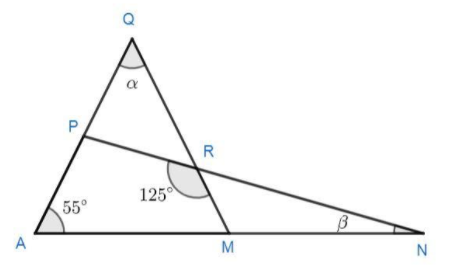In the diagram AMN, QRM and PRN are all straight lines. The value of $\alpha + \beta$ isVerified
147.6k+ views
Hint: Use the property of the triangle that the sum of the angles of the triangle is $180^\circ$in the triangle AQM, it gives:
$\angle AQM{\text{ }} + \angle QAM + \angle AMQ{\text{ }} = {\text{ }}180^\circ$
After that use the property of the sum of the linear pairs of angles, which says that if we have a pair of linear angles then their sum is$180^\circ$.

Complete step by step solution:
It is given in the problem that AMN, QRM, and PRN are all straight lines and we have to find the value of$\left( {\alpha + \beta } \right)$.
First, applying the angle sum property in triangle AQM,
We know that the sum of the angles in a triangle is always$180^\circ$. That is,
$\angle AQM{\text{ }} + \angle QAM + \angle AMQ{\text{ }} = {\text{ }}180^\circ$
Substitute the values of the given angles$\angle AQM = \alpha$and$\angle QAM = 55^\circ$into the equation.
$\alpha {\text{ }} + 55^\circ + \angle AMQ{\text{ }} = {\text{ }}180^\circ$
$\angle AMQ{\text{ }} = {\text{ }}180^\circ {\text{ }}-{\text{ }}55^\circ {\text{ }} - {\text{ }}\alpha$
On simplifying the above equation, we get
$\angle AMQ = 125^\circ {\text{ }} - {\text{ }}\alpha$
As we have given that AMN is a straight line, we know that the linear pair of angles have the sum$180^\circ$, then
$\angle$AMQ + $\angle$RMN = 180°
Now, substitute the values into the equation:
$\;125^\circ {\text{ }}-{\text{ }}\alpha {\text{ }} + \angle RMN{\text{ }} = {\text{ }}180^\circ$
$\angle RMN{\text{ }} = {\text{ }}55^\circ {\text{ }} + {\text{ }}\alpha$
It is also given to us in the problem that PRN is a straight line, so we know that the linear pair of angles have the sum $180^\circ$, then
$\angle PRM{\text{ }} + \angle MRN{\text{ }} = {\text{ }}180^\circ$
$125^\circ {\text{ }} + \angle MRN{\text{ }} = {\text{ }}180^\circ$
$\angle MRN{\text{ }} = {\text{ }}55^\circ$
We again use the property of the sum of the angles of the triangle MRN. Then we have,
$\angle MRN{\text{ }} + \angle RMN + \angle RNM{\text{ }} = {\text{ }}180^\circ$
Substitute the values into the equation:
$55^\circ + 55^\circ + \alpha + \beta = 180^\circ$
$\alpha + \beta = 70^\circ$

$\therefore$The required sum of angles have the value$\alpha + \beta = 70^\circ$.

Note:
Try to find all the angles of the triangle RMN in terms of $\alpha$using the angle sum property and linear pair of angles property of the triangle.